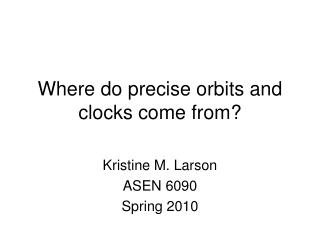DownloadDownload PresentationWhere do precise orbits and clocks come from?

# Where do precise orbits and clocks come from?

Télécharger la présentation## Where do precise orbits and clocks come from?

- - - - - - - - - - - - - - - - - - - - - - - - - - - E N D - - - - - - - - - - - - - - - - - - - - - - - - - - -
##### Presentation Transcript

1. Where do precise orbits and clocks come from? Kristine M. Larson ASEN 6090 Spring 2010

2. Starter question: where do broadcast orbits and clocks come from? • Always harder to predict orbits than to calculate them. Broadcast orbits and clocks are predictions. • DoD tracking network (with real-time telemetry). Positions of sites known “well.” • Real-time data analysis • Simple models (note e.g. clock t and t^2 terms) • Pseudorange data? • No mandate for cm-level precision.

3. Which broadcast ephemeris? • Modifications in the 1990s included updating solar radiation pressure models, satellite mass, ground station coordinates, and process noise covariance values.

4. How do we evaluate orbit accuracy? • How do we evaluate orbit precision? • The same questions exist for clocks.

5. The code you are using • Need Keplerian elements and fudge factors from Nav message. • The code solves Kepler’s equations. • Officially, only GM and constant Earth rotation are used. • Applies multiple corrections (delta terms). Remember: the message only has to be good for 2 hours. • Last bit: Rotates from Earth-centered Inertial to Earth-centered Earth-fixed.

6. Ephemeris Code u = phi + cuc*cos(2*phi)+cus*sin(2*phi); r = A*(1-ecc*cos(E)) + crc*cos(2*phi)+crs*sin(2*phi); i = i0+idot*tk + cic*cos(2*phi)+cis*sin(2*phi); x1 = cos(u)*r; y1 = sin(u)*r; satp(1,1) = x1*cos(Omega)-y1*cos(i)*sin(Omega); satp(2,1) = x1*sin(Omega)+y1*cos(i)*cos(Omega); satp(3,1) = y1*sin(i);

7. For highest accuracy • Most of what was discussed in the previous page is wrong or sub-optimal in some sense. • More terms of the Earth’s gravity field are needed. • Tracking site positions are much better known. • Better models are needed for the space and ground segments. • Earth rotation is not constant. • Earth’s pole of rotation changes. • Use carrier phase data (5 mm precision instead of 50 cm precision).

8. How do you know what they did to produce your precise orbits (i.e. the sp3 file)? Look at the IGS website to find out! http://igscb.jpl.nasa.gov/igscb/center/analysis/esa.acn http://igscb.jpl.nasa.gov/igscb/center/analysis/jpl.acn

9. Draw the frame on the board (last time we talked about the “ruler”)

10. What is a ppb?

11. One year of broadcast orbits +- 40 m +- 20 m +2/-6 m Igs orbits being treated as truth

12. Block II

13. Block IIR

14. apples and oranges

15. Why would we expect them to be the same?

16. Does everything get better with time?

17. radial/cross/along 0 - 10 m

18. Things to keep in mind • Evaluating orbit precision (and accuracy) is a moving target on both sides - broadcast orbits are changing (for reasons that may have nothing to do with improvements to physical models) • Civilian (IGS) precise orbits are also changing for a variety of reasons.

19. Broadcast-precise model differences • J2 (FF) • Other harmonics (FF) • Sun/Moon (FF) • Earth tides (FF) • Ocean tides • Solar pressure (big effect!) • Albedo pressure We’ll talk about these model changes on Monday

20. Keep in mind • There is more than one kind of IGS orbit • Precise (available after ~week) • Rapid (within 24 hours) • Ultra-rapid (real-time)

21. IGS (precise) orbits • Implement these model changes. • Conventions are required (as we talked about last time) • Individual centers can do research to evaluate the efficacy of other models. • When significant model changes are made, what happens to the IGS precise orbit products?

22. Other issues: Not all analysis centers are created equal

23. The IGS also produces Clocks What is a ns?

24. Frame Parameters

25. Variability by analysis center

26. How good are the IGS Ultra Rapids?

27. Monday, January 25 • OH: M1-2:30 and F 2-3:30 and MWF by appt. • Talk about homework 1 • Questions about homework 2 • Precise reference frames - which leads us into precise orbit determination

28. ECI to ECEF Earth Centered Inertial to Earth Centered Earth Fixed And vice versa

29. Ways to think about the frame • We are really talking about XYZ. And goals are all relative to 1mm. • Rotation axis in space • Rotation axis in the “Earth” • How fast does the Earth rotate. • Complication: tectonic plates moving on the surface of the Earth. • How are we going to realize the “Earth?” (we’ll talk about the link to space as well).

30. Realizing the Frame • Measurements on the Earth to: • Satellites using lasers • Quasars using radio telescopes • Satellites, radio freq., ranges (GPS, Glonass) • Satellites, doppler • Some laser measurements to the moon - but not very important • Once we know where “things” are, we fix the coordinates of these sites, and estimate other things (such as orbits of satellites)

31. The frame • Is updated regularly (every 2-3 years). • It attempts to combine the “best” of all techniques. • ITRF (International Terrestrial Reference Frame) is the ECEF part. • It is not a frame for purposes of comparison- its goal is to correctly define the Earth’s crust wrt to XYZ.

32. How is the frame distributed? • Positions at an epoch and velocities. Is this adequate? What kind of discontinuities exist in time series, and why?

33. 2004-2010

34. http://pboweb.unavco.org/shared/scripts/stations/?checkkey=TPW2http://pboweb.unavco.org/shared/scripts/stations/?checkkey=TPW2

35. Discontinuities & non-linearities are an ongoing problem in frame realization

36. Why SLR ? Why not GPS? Is this a problem?

37. Colocation • Multiple measurement systems don’t do much for you unless you have some of them in the same place.

38. Show zuheir’s ITRF2008 plot

39. Where do these come from?